5G - Propagation Model                                                          Home : www.sharetechnote.com Propagation Modeling/Channel Impulse Response   The purpose of this page is to describe about how the property of the milimeter wave signal varies as it propagate through the physical media (i.e channel, e.g, air, buldings, trees etc). The modeling of a signal going through a channel is called CIR (Channel Impulse Response) and it is the most fundamental investigation of any wireless communication techologies (If you are not familiar with the term 'Impulse response', refer to Impulse respone page). From this research and investigation, we will eventually obtain more model like Fading Channel Model and MIMO channel model.   This page is mainly motivated by the recent announcement from NYU who decided to share tons of their work for many years and real measurement data, mathematical model as an opensource in Mar 2016 (Refer to Open Source Downloadable 5G Channel Simulator software). This is extremly greatful and thankful, and will definitely invaluable contribution to the whole industry. I think many of you have already seen many presentation slides and papers from this team, and with the opensource you will get the chance to understand those slides/papers as you did all those test by yourself. Reading some of the papers written by the team, I could sense that they have really willingness to share what they have done and trying to help others. Even from very short and very formal papers published in popular journal, you would see they desribe things in very practical way. I strongly recommend you to look into this and search various documents from the team (I put some of them at the reference section).   I will take those material/papers from NYU as a skeleton of this page and keep extending as I learn more from various other sources.     Factors for Channel Impulse Model.   What are the factors defining the nature of the radio channel ? There are many factors, but if I pick only a few important factors, I would list as follows. i) Path Loss between the transmitter and reciever. This defines how much energy gets lost while the signal is going through the channel (media) ii) Delay Spread. This indicates how much the signal get dispersed in time domain (in terms of reception timing at Rx Antenna). Refer to Delay Spread page for the detailed concept. iii) Angle of Arrival. This indicates how the nature of the received signal (mostly the received power and phase) changes with angle of reciever antenna with a specific reference point. You may add more items to the list, but I think these three would be the most dominant factors defining the channel impulse model. These three factors can be combined into one frame as illustrated below. and I will describe more details on each of the factors.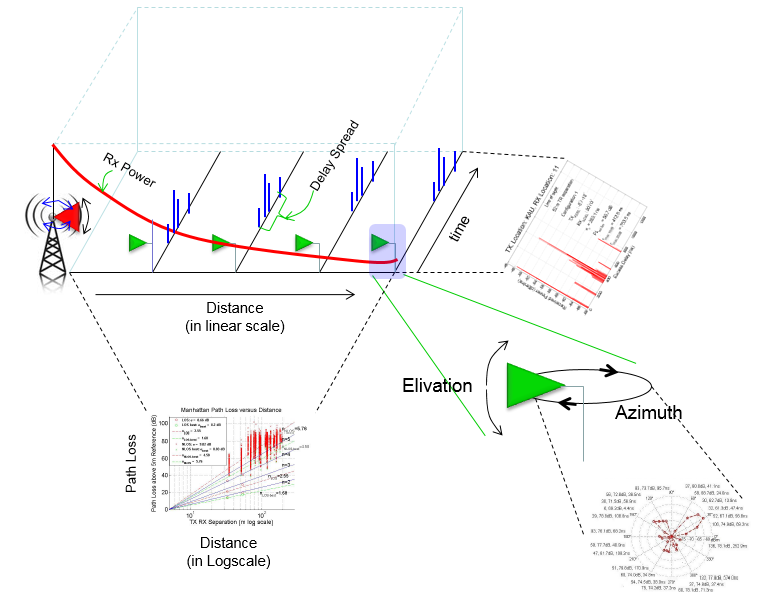Path Loss Modeling   Path Loss between a Transmitter and Reciever can be best described by a plot. But you may see slightly different form of the plots from different papers and text books and sometimes those different representation may confuse you. So, it would be good to think of all the possible types of graph/plots before you reading papers and textbooks. I put the four different possible types which basically indicate the same physical properties but they are plotted a little different ways.   I think < A > would be the most straightfoward and intuitive representation. In this, the vertical axis represents the power measured by the receiver antenna and the horizontal axis represents the distance between Tx and Rx antenna in linear scale. By common sense and intuition, you would easily guess the received power will decrease as the distance gets larger. But how much the power get decreased ? Does it decrease by linear (straight line) fashion ? or some non linear (curve) fashion ? By high school physics, you may guess the power will decrease in anti-proportion to the distance squared. For more specifically, refer to Path Loss Model in Free Space.  Now you can sense that the recieved power with distance would be something as in < A >. < B > is telling the exactly same thing as < A > but you see the straight line not the curve. Why ? it is because the scale of the horizontal axis is Log scale. By nature (at least to me), Log scale does not looks natural.. but in some case converting it to linear scale is helpful to convert a nonlinear plot to linear plot and can process more easily in terms of statistics. Especially when you process measured data, linear fitting would be much easier than a curve fitting. So in many paper or textbook, you would see the plots represented as in  < B > < C > represents Path Loss with the distance between Tx and Rx. If you compare < A > and < C >, you would notice that they are complimentary. It is common sense. As Path Loss goes higher, the received power would goes lower. With the distance represented in linear scale, you see the Path Loss is plotted in non-linear (curve) pattern. < D > is telling the exactly same thing as < C > but you see the straight line. This straight line is obtained by converting the horizontal scale (distance) to log scale.Now let's look into the examples from real papers. One paper from NYU (Reference ,) shows following plot. This plot is based on type < D > described above. What you need to see from this graph is that the degree of Pass Loss change (Path Loss increase with the distance between Tx and Rx) changes depending on various situations.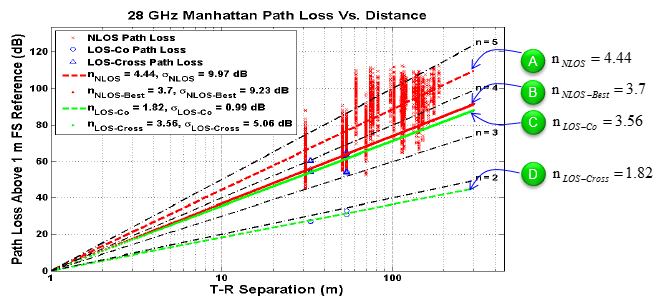< From Reference   >   Once you have the plots from the measured data as shown above, you may be able to derive a certain mathematical model that explains the measured data. The mathematical model for the Path Loss for this plot is represented as shown below (This model came from Reference  and Matlab source code in Reference ). For the textbook style description/derivation of this model, refer to Path Loss Model in Real Situation : Shadowing.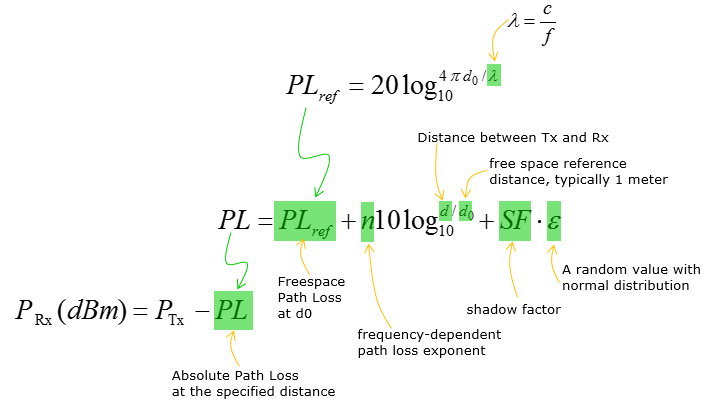Delay Spread   A property that represents a time domain nature of a channel is Delay Spread (See Delay Spread page if you are not familiar with the concept).   Following (Reference ) is the measurement of Delay Spread performed at a location 52 m away from the transmitter. As you see here, tau max (20 dB) is 735.5 ns which is equivalent to 226 m travel path. It implies that it is high degree of multi path environment.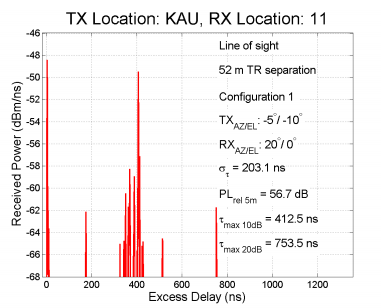< From Reference  >   From Reference , you can get following plot showing the delay spread with various reciever locations.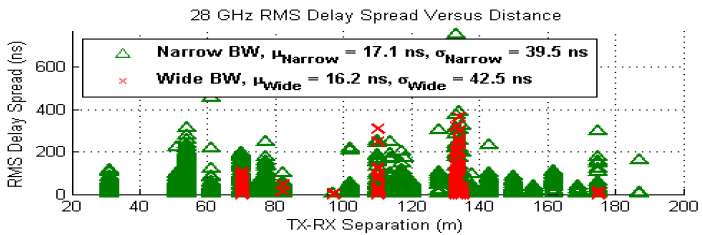< From Reference  >     Angle of Arrival   Another important property that shows the nature of a radio channel is Angle of Arrival. This can be obtained by measuring the recieived power while rotating the reciever antenna when the direction of transmission antenna is fixed. One example measurement from Reference  is as follows. You may notice there are several distinctive lobes here. This implies that there are several multipath that can play dominant roles in received signal.< From Reference 3 >   Following (Reference ) may not be directly related to 5G wave propogation, but I posted it here since it would give you a good idea on how to interpret the AoA plot shown above.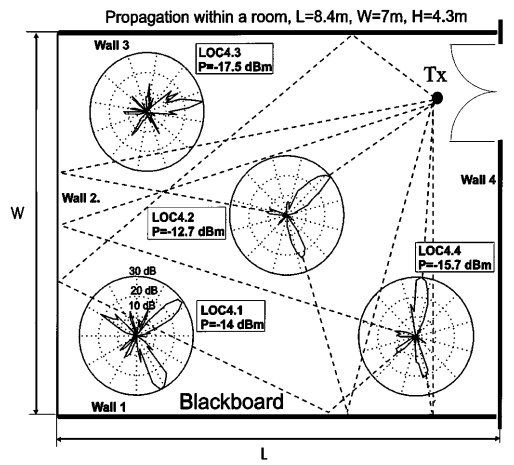< From Reference  >     Reference           NYU WIRELESS 5G Millimeter Wave Statistical Channel Model Suitable for 3GPP         and Academic/Industrial Simulations            Using Steerable Beam Antennas in New York City Yaniv Azar, George N. Wong, Kevin Wang, Rimma Mayzus, Jocelyn K. Schulz, Hang Zhao, Felix Gutierrez, Jr., DuckDong Hwang, Theodore S. Rappaport NYU WIRELESS Polytechnic Institute of New York University, Brooklyn, NY 11201   Professor Theodore (Ted) S. Rappaport NYU WIRELESS New York University School of Engineering By Mary Miniuk Thesis Submitted to the Faculty of the Virginia Polytechnic Institute and State University in partial fulfillment of the requirements for the degree of   MASTER OF SCIENCE in Electrical Engineering    Spatial and Temporal Characteristics of 60-GHz Indoor Channels by Hao Xu, Member, IEEE, Vikas Kukshya, Member, IEEE, and Theodore S. Rappaport, Fellow, IEEE IEEE JOURNAL ON SELECTED AREAS IN COMMUNICATIONS, VOL. 20, NO. 3, APRIL 2002